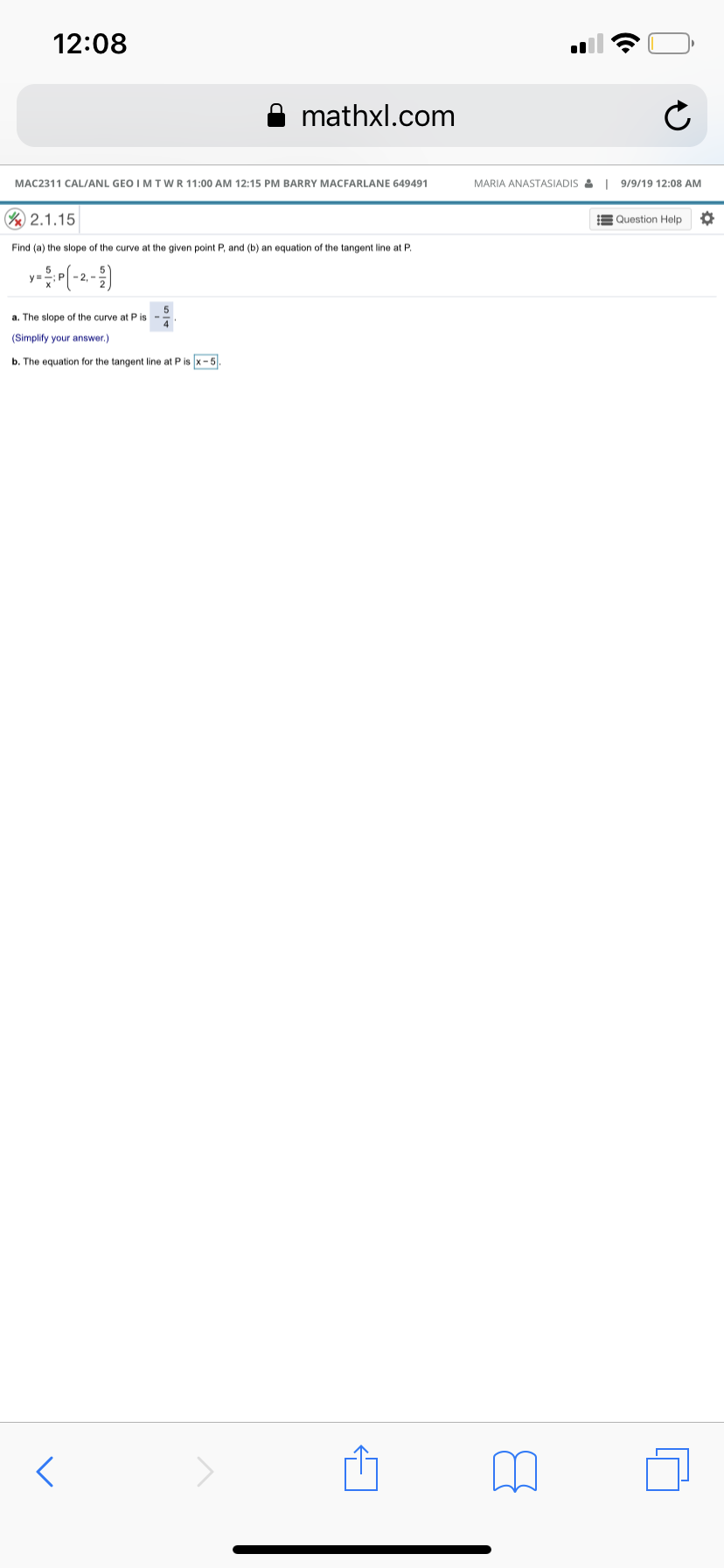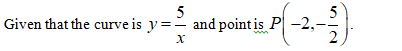# 12:08mathxl.comMARIA ANASTASIADIS&I 9/9/19 12:08 AMMAC2311 CAL/ANL GEO I M TWR11:00 AM 12:15 PM BARRY MACFARLANE 649491V 2.1.15Question HelpFind (a) the slope of the curve at the given point P, and (b) an equation of the tangent line at P.2.5a. The slope of the curve at P is4(Simplify your answer.)b. The equation for the tangent line at P is x -5

Question
12 views

Can you help me step by step with this problem?help_outlineImage Transcriptionclose12:08 mathxl.com MARIA ANASTASIADIS&I 9/9/19 12:08 AM MAC2311 CAL/ANL GEO I M TWR11:00 AM 12:15 PM BARRY MACFARLANE 649491 V 2.1.15 Question Help Find (a) the slope of the curve at the given point P, and (b) an equation of the tangent line at P. 2. 5 a. The slope of the curve at P is 4 (Simplify your answer.) b. The equation for the tangent line at P is x -5 fullscreen
check_circle

Step 1Step 2
1. a) The slope of the curve is
Step 3

b) The equation of th...

### Want to see the full answer?

See Solution

#### Want to see this answer and more?

Solutions are written by subject experts who are available 24/7. Questions are typically answered within 1 hour.*

See Solution
*Response times may vary by subject and question.
Tagged in

### Calculus